# 比例谐振（PR）控制器的学习过程记录

12 篇文章 36 订阅

0、前言

1、PR控制器和PI控制器对比

1.1 传递函数表达式对比

1.2 波特图对比

2、离散化预备知识

2.1 离散化表达式

2.2 离散化方法

2.3 离散化练习题

3. 使用Matlab离散PR控制器

4、逆变器仿真模型中使用PR闭环控制器

## 0、前言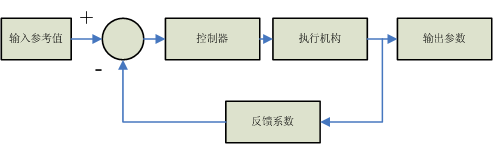## 1、PR控制器和PI控制器对比

### 1.2 波特图对比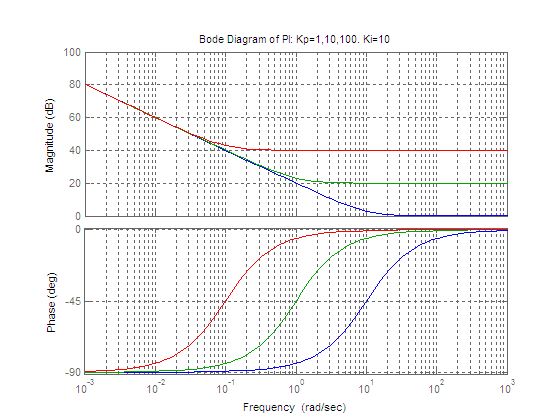% this is Matlab code:
Ki=10;
Kp=1;
PIs1=tf([Kp,Ki],[1,0])
Kp=10;
PIs2=tf([Kp,Ki],[1,0])
Kp=100;
PIs3=tf([Kp,Ki],[1,0])
bode(PIs1,PIs2,PIs3)
title('Bode Diagram of PI: Kp=1,10,100. Ki=10')
grid on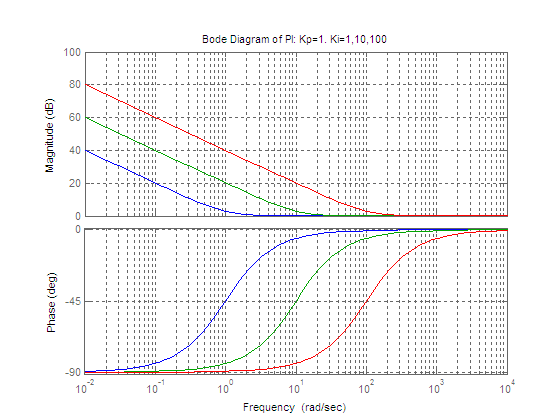Kp=1
Ki=1
PIs1=tf([Kp,Ki],[1,0])
Ki=10
PIs2=tf([Kp,Ki],[1,0])
Ki=100
PIs3=tf([Kp,Ki],[1,0])
figure(2)
bode(PIs1,PIs2,PIs3)
grid on
title('Bode Diagram of PI: Kp=1. Ki=1,10,100')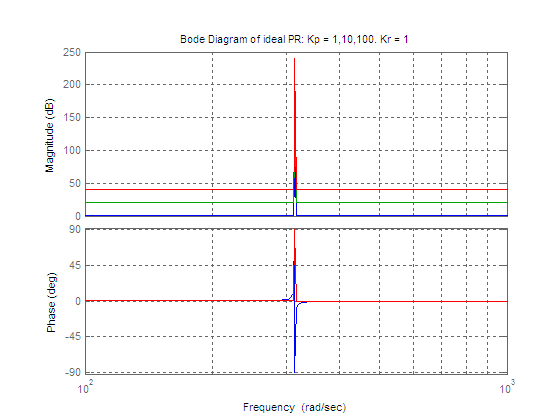Kr=1;
Kp=1;
PR_ideal1 = Kp + tf([Kr,0],[1,0,wo^2])
Kp=10;
PR_ideal2 = Kp + tf([Kr,0],[1,0,wo^2])
Kp=100;
PR_ideal3 = Kp + tf([Kr,0],[1,0,wo^2])
bode(PR_ideal1,PR_ideal2,PR_ideal3)
grid on
title('Bode Diagram of ideal PR: Kp = 1,10,100. Kr = 1')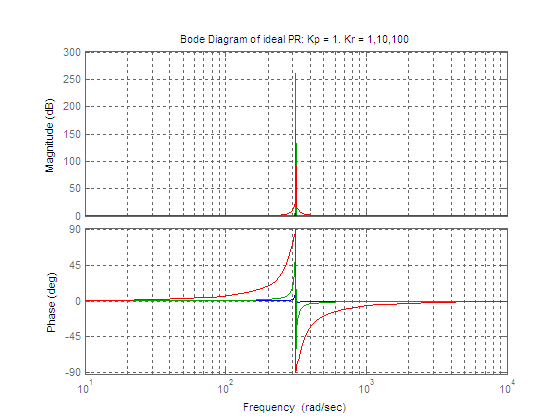Kp=1;
Kr=1;
PR_ideal1 = Kp + tf([Kr,0],[1,0,wo^2])
Kr=10;
PR_ideal2 = Kp + tf([Kr,0],[1,0,wo^2])
Kr=100;
PR_ideal3 = Kp + tf([Kr,0],[1,0,wo^2])
figure(4)
bode(PR_ideal1,PR_ideal2,PR_ideal3)
grid on
title('Bode Diagram of ideal PR: Kp = 1. Kr = 1,10,100')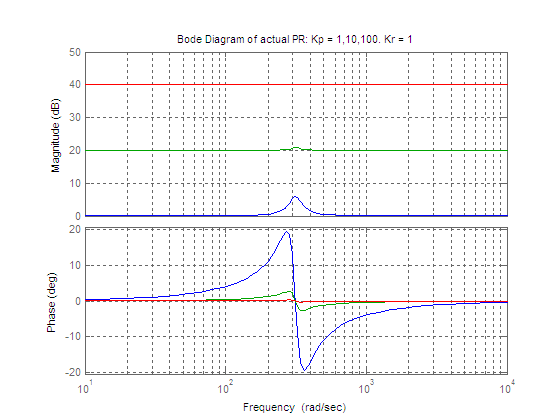Kr=1;
Kp=1;
PR1=Kp+tf([2*Kr*wc,0],[1,2*wc,wo^2])
Kp=10;
PR2=Kp+tf([2*Kr*wc,0],[1,2*wc,wo^2])
Kp=100;
PR3=Kp+tf([2*Kr*wc,0],[1,2*wc,wo^2])
bode(PR1,PR2,PR3)
grid on
title('Bode Diagram of actual PR: Kp = 1,10,100. Kr = 1')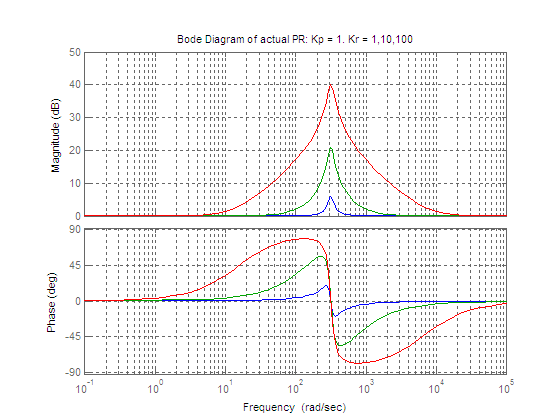Kp=1;
Kr=1;
PR1=Kp+tf([2*Kr*wc,0],[1,2*wc,wo^2])
Kr=10;
PR2=Kp+tf([2*Kr*wc,0],[1,2*wc,wo^2])
Kr=100;
PR3=Kp+tf([2*Kr*wc,0],[1,2*wc,wo^2])
bode(PR1,PR2,PR3)
grid on
title('Bode Diagram of actual PR: Kp = 1. Kr = 1,10,100')

PI和PR的Kp作用类似，都是增大开环增益，增加控制精度。

Ki和Kr作用类似：降低系统稳态误差。

## 2、离散化预备知识

### 2.2 离散化方法

 Zero-order hold First-order hold Forward Euler Backward Euler Trapezoid(Tustin) Tustin with pre-warping Zero-pole matching Impulse invariant

### 2.3 离散化练习题

 Kp=1;Ki=2;Kd=3;Ts=1e-3;
PID=tf([Kd,Kp,Ki],[1,0])
c2d(PID,1e-3,'tustin')

Transfer function:
6001 z^2 - 1.2e004 z + 5999
---------------------------
z^2 - 1

Sampling time: 0.001


SYSD = C2D(SYSC,TS,METHOD) computes a discrete-time model SYSD with
sampling time TS that approximates the continuous-time model SYSC.
The string METHOD selects the discretization method among the following:
'zoh'       Zero-order hold on the inputs
'foh'       Linear interpolation of inputs
'impulse'   Impulse-invariant discretization
'tustin'    Bilinear (Tustin) approximation.
'matched'   Matched pole-zero method (for SISO systems only).
The default is 'zoh' when METHOD is omitted.

## 3. 使用Matlab离散PR控制器

Kp=1;
Kr=10;
wc=2*pi*5;
wo=2*pi*50;
PRs=Kp+tf([2*Kr*wc,0],[1,2*wc,wo^2])
PRz = c2d(PRs,1e-3,'tustin')


Transfer function:
1.297 z^2 - 1.847 z + 0.643
---------------------------
z^2 - 1.847 z + 0.9405

Sampling time: 0.001

y_k - 1.847*y_k1 + 0.9405*y_k2 = 1.297*u_k - 1.847*u_k1 +0.643*u_k2;

y_k = 1.297*u_k - 1.847*u_k1 +0.643*u_k2 + 1.847*y_k1 - 0.9405*y_k2 ;

## 4、逆变器仿真模型中使用PR闭环控制器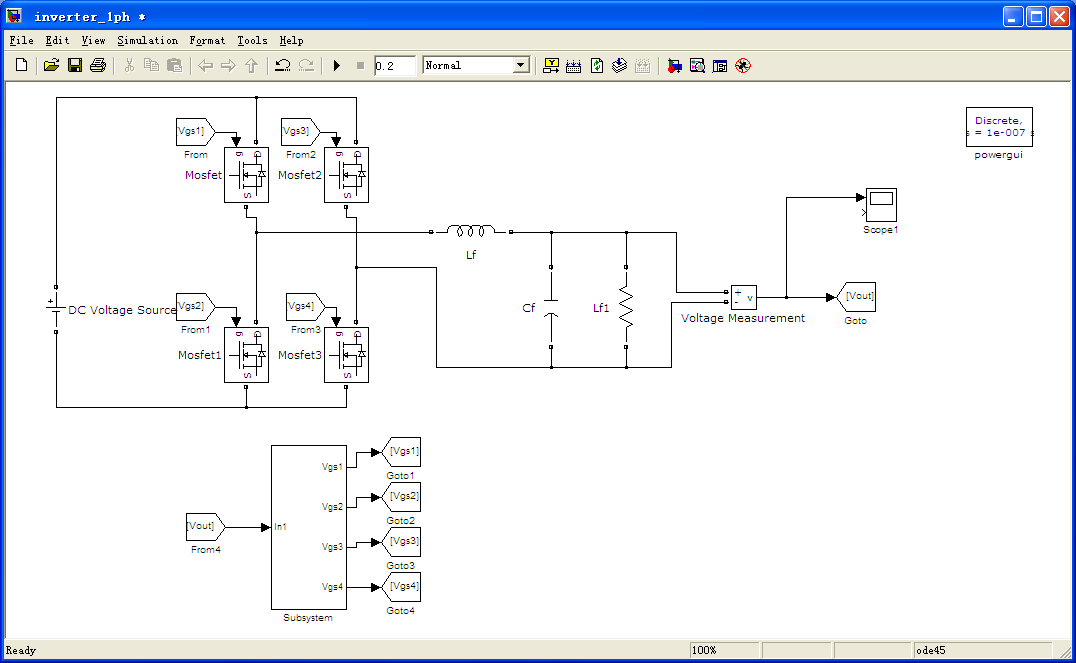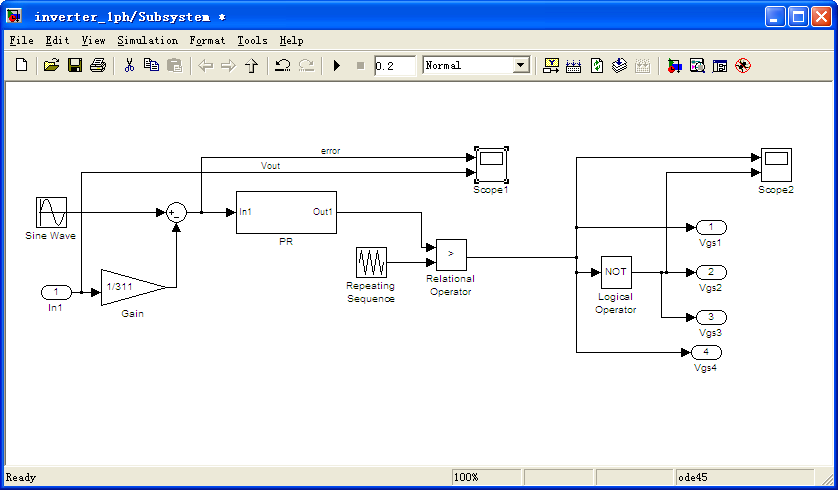PR控制器实现框图：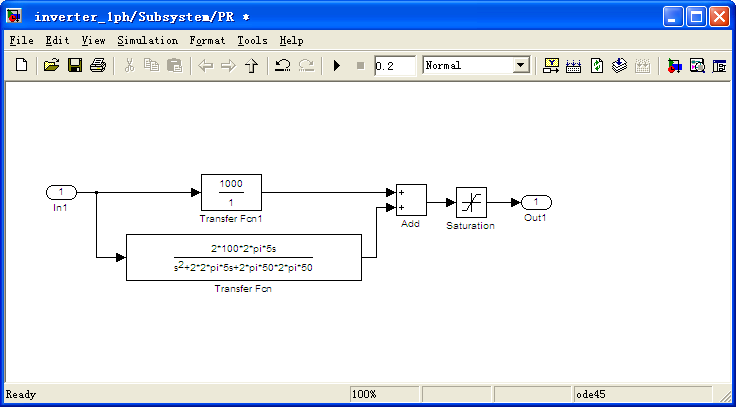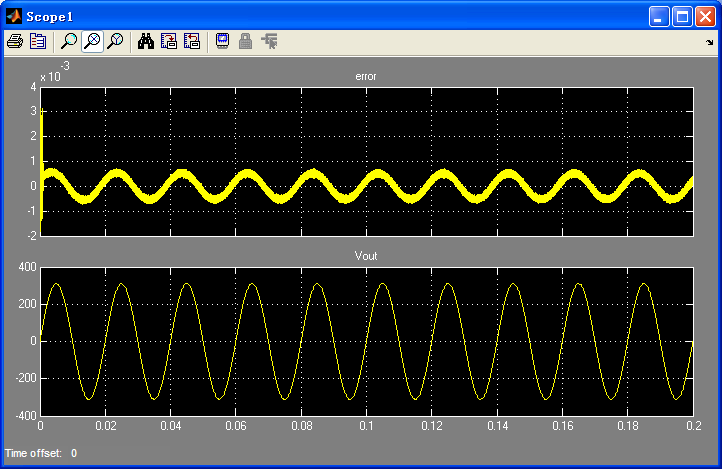04-26
03-2402-041187
03-131万+
03-31
03-24693
05-01536
05-20416
08-18
07-032794
05-281516
03-07426
05-27829
12-26801
03-312569
09-278589点击重新获取扫码支付余额充值